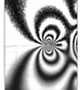Lecture Notes Part I. Elasticity 1. Introduction 2. Tensors 3. Hooke's Law 4. Fundamental Equations of Elasticity 5. 2D Elasticity 6. Rectangular Beam 7. Fourier Series and Transform 8. Fourier Solution 9. Half Space 10. Contact 11. Polar Coordinates 12. Wedge and Notch Part II. Plasticity 13. Fundamental Equations of Plasticity 14. Graphical Representations 15. Tension and Shear 16. Plastic Bending 18. Hardening Law 20. Crystal Plasticity Part III. Fracture 22. Slit-like Crack 23. Energy Release Rate 24. Linear Elastic Fracture Mechanics 25. Elastic Plastic Fracture Mechanics 26. Fatigue

ME 340 Elasticity and InelasticityThe goal of the class is to provide an introduction to the theory of elasticity, plasticity and fracture and their applications. Elasticity: stress function approach to solve 2D problems and Greenżs function in 3D; applications to contact problems. Plasticity: yield surface, associative flow rule, strain hardening models; and applications to plastic bending, torsion and pressure vessels. Fracture: linear elastic fracture mechanics, J-integral, plastic zone in front of crack tip; applications to brittle fracture and fatigue crack growth. Computer programming in Matlab is used to aid analytic derivation and numerical solutions. Textbook: J. R. Barber, Elasticity, 3rd ed. Springer (2010); T. L. Anderson, Fracture Mechanics, 3rd ed. Taylor & Francis (2005).

All Notes in One PDF File